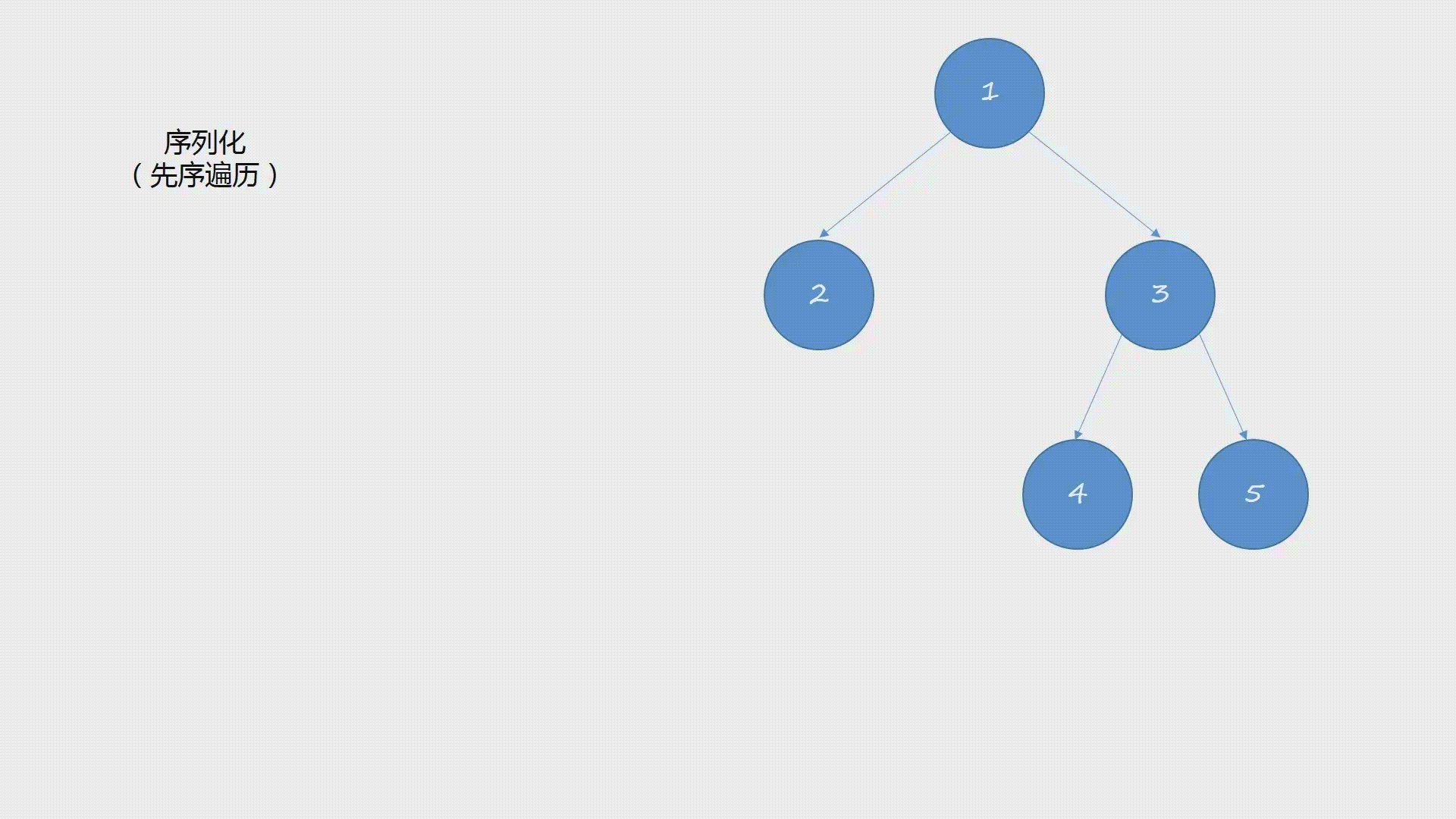# LeetCode 297. Serialization and deserialization of binary trees | Python

Posted Jun 16, 20204 min read

### 297. Serialization and deserialization of binary trees

Source of the question:LeetCode [ https://leetcode-cn.com/problems/serialize-and-deserialize-binary-tree] ( https://leetcode-cn.com/problems/serialize-and-deserialize- binary-tree)

#### Title

Serialization is the operation of converting a data structure or object into consecutive bits, and then the converted data can be stored in a file or memory, and can also be transmitted to another computer environment through the network, and reconstructed in the opposite way Get the original data.

Please design an algorithm to realize the serialization and deserialization of the binary tree. There is no restriction on the execution logic of your sequence/deserialization algorithm. You only need to ensure that a binary tree can be serialized into a string and deserialize the string into the original tree structure.

Example:

You can put the following binary tree:

``````    1
/\
twenty three
/\
4 5

Serialized as "[1,2,3,null,null,4,5]"``````

Hint: This is consistent with the current method used by LeetCode. For details, please refer to the format of LeetCode serialized binary tree. You do not have to adopt this method, you can also use other methods to solve this problem.

Note: Do not use class members/globals/static variables to store state, your serialization and deserialization algorithms should be stateless.

#### Problem solving ideas

Thinking:depth first search

According to the topic, we can learn. In fact, binary tree serialization is to save the binary tree as a string in a certain format according to a certain traversal method. In this article, we use the method of depth first search to achieve this goal.

Here, the idea of depth-first search we use is implemented by recursion. In this process, we use the method of pre-order traversal to solve.

When we use recursion, we only need to focus on a single node, and leave the rest to the recursive implementation. The reason for using preorder traversal is that the access order of preorder traversal is to first visit the root node, then traverse the left subtree, and finally traverse the right subtree. This way we can directly locate the root node when we first deserialize.

There is a need to pay attention to here, when encountering a null node, to be identified. In this way, it can be distinguished that it is a null node when it is deserialized.

The following uses the sequential traversal method, with the help of Example 1, to illustrate the serialization process in a graphical form:In the above illustration, the serialized character is 1 starting from the root node 1 according to the access sequence of the first traversal. Then traverse the left subtree, the root node of the left subtree is 2, and the serialized characters are 1, 2,. At this time, starting from 2, visit the left node(1, 2, null,) and the right node(1, 2, null, null). In this case, null is the aforementioned symbol marked null. This returns to the root node and accesses the right subtree. The access sequence is also traversed in order, and the final serialized string results are:1, 2, null, null, 3, 4, null, null, 5, null, null,.

As for how to deserialize? According to the rules of the access sequence of traversing first, we traverse this serialized string from left to right:

• When the current element is null, it is an empty tree;
• When the above situation does not exist, first parse the left subtree, and then parse the right subtree

The specific implementation code is as follows.

#### Code

``````# Definition for a binary tree node.
# class TreeNode(object):
# def __init__(self, x):
# self.val = x
# self.left = None
# self.right = None

class Codec:

def serialize(self, root):
"""Encodes a tree to a single string.

:type root:TreeNode
:rtype:str
"""
if root == None:
return'null,'
left_serialize = self.serialize(root.left)
right_serialize = self.serialize(root.right)
return str(root.val) +',' + left_serialize + right_serialize

def deserialize(self, data):
"""Decodes your encoded data to tree.

:type data:str
:rtype:TreeNode
"""
def dfs(queue):
val = queue.popleft()
if val =='null':
return None
node = TreeNode(val)
node.left = dfs(queue)
node.right = dfs(queue)
return node

from collections import deque

queue = deque(data.split(','))

return dfs(queue)

# Your Codec object will be instantiated and called as such:
# codec = Codec()
# codec.deserialize(codec.serialize(root))``````

#### Results#### to sum up

• First understand that binary tree serialization is actually based on a certain traversal method, and the result is saved as a string in a certain format.

• Adopt the method of dfs(bfs can also be used), serialize the binary tree according to the access method of traversal in order. The method of pre-order traversal used here is also convenient to quickly locate the root node during deserialization. Because the access sequence of the first traversal is:first visit the root node, then traverse the left subtree, and finally traverse the right node.

• When serializing, when it encounters None, it needs to be identified, so that it can be distinguished as None when it is deserialized, which is an empty tree.

• Deserialization, according to the access rule of traversing first, split the previously serialized string, and traverse from left to right:

• When the element is null, it means an empty tree;
• Otherwise, the left subtree is parsed first, and then the right subtree is parsed.